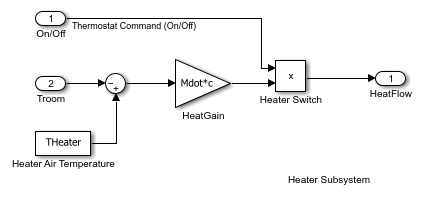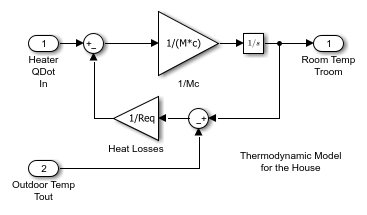Documentation

## Thermal Model of a House

This example shows how to use Simulink® to create the thermal model of a house. This system models the outdoor environment, the thermal characteristics of the house, and the house heating system.

The sldemo_househeat_data.m file initializes data in the model workspace. To make changes, you can edit the model workspace directly or edit the file and re-load the model workspace. To view the model workspace, select View > Model Explorer from the Simulink editor.

### Opening the Model

Open the sldemo_househeat modelFigure 1: The House Heating Model

### Model Initialization

This model calculates heating costs for a generic house. When the model is opened, it loads the information about the house from the sldemo_househeat_data.m file. The file does the following:

• Defines the house geometry (size, number of windows)

• Specifies the thermal properties of house materials

• Calculates the thermal resistance of the house

• Provides the heater characteristics (temperature of the hot air, flow-rate)

• Defines the cost of electricity (0.09\$/kWhr)

• Specifies the initial room temperature (20 deg. Celsius = 68 deg. Fahrenheit)

• Note: Time is given in units of hours. Certain quantities, like air flow-rate, are expressed per hour (not per second).

### Model Components

Set Point

"Set Point" is a constant block. It specifies the temperature that must be maintained indoors. It is 70 degrees Fahrenheit by default. Temperatures are given in Fahrenheit, but then are converted to Celsius to perform the calculations.

Thermostat

"Thermostat" is a subsystem that contains a Relay block. The thermostat allows fluctuations of 5 degrees Fahrenheit above or below the desired room temperature. If air temperature drops below 65 degrees Fahrenheit, the thermostat turns on the heater. See the thermostat subsystem below.

Open the Thermostat subsystemFigure 2: The "Thermostat" Subsystem

Heater

"Heater" is a subsystem that has a constant air flow rate, "Mdot", which is specified in the sldemo_househeat_data.m file. The thermostat signal turns the heater on or off. When the heater is on, it blows hot air at temperature THeater (50 degrees Celsius = 122 degrees Fahrenheit by default) at a constant flow rate of Mdot (1kg/sec = 3600kg/hr by default). The heat flow into the room is expressed by the Equation 1.

Equation 1

``
``
``
``
``
``

Open the Heater subsystemFigure 3: The Heater Subsystem

Cost Calculator

"Cost Calculator" is a Gain block. "Cost Calculator" integrates the heat flow over time and multiplies it by the energy cost. The cost of heating is plotted in the "PlotResults" scope.

House

"House" is a subsystem that calculates room temperature variations. It takes into consideration the heat flow from the heater and heat losses to the environment. Heat losses and the temperature time derivative are expressed by Equation 2.

Equation 2

``
``
``
``

Open the House subsystemFigure 4: The House Subsystem

Modeling the Environment

We model the environment as a heat sink with infinite heat capacity and time varying temperature Tout. The constant block "Avg Outdoor Temp" specifies the average air temperature outdoors. The "Daily Temp Variation" Sine Wave block generates daily temperature fluctuations of outdoor temperature. Vary these parameters and see how they affect the heating costs.

### Running the Simulation and Visualizing the Results

Run the simulation and visualize the results. Open the "PlotResults" scope to visualize the results. The heat cost and indoor versus outdoor temperatures are plotted on the scope. The temperature outdoor varies sinusoidally, whereas the indoors temperature is maintained within 5 degrees Fahrenheit of "Set Point". Time axis is labeled in hours.Figure 5: Simulation results (time axis labeled in hours)

According to this model, it would cost around \$30 to heat the house for two days. Try varying the parameters and observe the system response.

### Remarks

This particular model is designed to calculate the heating costs only. If the temperature of the outside air is higher than the room temperature, the room temperature will exceed the desired "Set Point".

You can modify this model to include an air conditioner. You can implement the air conditioner as a modified heater. To do this, add parameters like the following to sldemo_househeat_data.m.

• Cold air output

• Temperature of the stream from the air conditioner

• Air conditioner efficiency

You would also need to modify the thermostat to control both the air conditioner and the heater.